Courses

# Model Test Paper - 1

## 30 Questions MCQ Test Science Olympiad Class 6 | Model Test Paper - 1

Description
This mock test of Model Test Paper - 1 for Class 6 helps you for every Class 6 entrance exam. This contains 30 Multiple Choice Questions for Class 6 Model Test Paper - 1 (mcq) to study with solutions a complete question bank. The solved questions answers in this Model Test Paper - 1 quiz give you a good mix of easy questions and tough questions. Class 6 students definitely take this Model Test Paper - 1 exercise for a better result in the exam. You can find other Model Test Paper - 1 extra questions, long questions & short questions for Class 6 on EduRev as well by searching above.
QUESTION: 1

Solution:
QUESTION: 2

Solution:
QUESTION: 3

### The following image shows: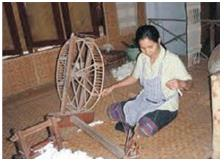Solution:
QUESTION: 4

Identify the fiber: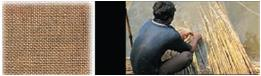Solution:
QUESTION: 5

Because of the crimp,_________________ fabrics have greater bulk than other textiles and retain air, which causes the product to retain heat.

Solution:
QUESTION: 6

Study the picture below and answer the questions that follow.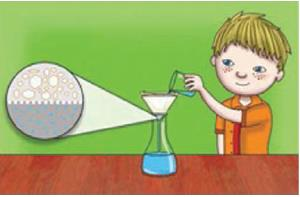Q. Which statement describe the picture correctly?

Solution:
QUESTION: 7

The classification chart below shows the properties of four different materials. Which materials is most suitable for making raincoat?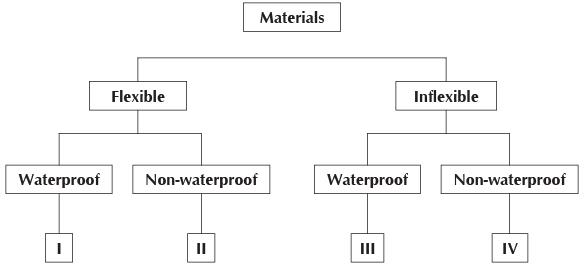Solution:
QUESTION: 8

The chart below shows how some objects are grouped into two groups, X and Y.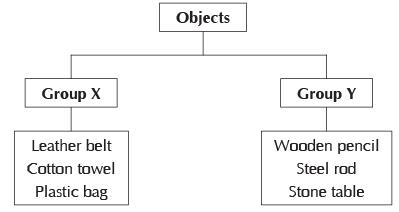Q. Which of the following headings best describe the two groups?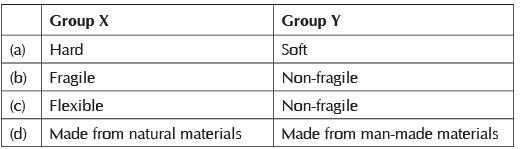Solution:
QUESTION: 9

Choose the substance that shows sublimation:

Solution:
QUESTION: 10

Cooking of rice is an example of:

Solution:
QUESTION: 11

Iron rusts because:

Solution:
QUESTION: 12

The reactions which are accompanied by production of heat are called .

Solution:

Some reactions produce heat and are called exothermic reactions and others may require heat to enable the reaction to occur, which are called endothermic reactions.

QUESTION: 13

Phyllotaxy is the best arrangement of leaves on the stem. Identify the three types of phyllotaxy shown in the figure given below: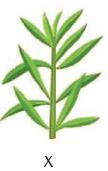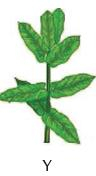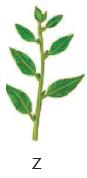Solution:
QUESTION: 14

Read the features of a plant as given below.
1. Roots are much shorter in size.
2. Stems are generally long and narrow.
3. Stems possess air spaces.
4. They have waxy upper surface.

Q. To which of these habitats does this plant belong?

Solution:
QUESTION: 15

The organisms which are smaller than bacteria are:

Solution:

A virus is a small infectious agent, smaller than bacteria, that replicates only inside the living cells of other organisms. Viruses can infect all types of life forms, from animals and plants to microorganisms, including bacteria and archaea.

QUESTION: 16

One centimeter on a scale is divided into 20 equal divisions. The least count of this scales is:

Solution:

According to the question 1 cm scale is divided into 20 equal divisions.
20 division = 1 cm
1 division = 1/20cm
= 0.05 cm
= 0.05×10 mm
= 0.5 mm
So, the least count of the scale is 0.5 mm

QUESTION: 17

The motion of the arm of a soldier marching along the road is:

Solution:
QUESTION: 18

Which of the following statements should be followed while using a symbol for a unit of a physical quantity?

Solution:
QUESTION: 19

A solid, transparent sphere has a small, opaque center. When observed from outside, the apparent position of the dot will be:

Solution:
QUESTION: 20

At 8 a.m., the shadow of Ananya’s flat was as shown below.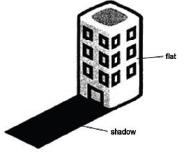Q. Which one of the following diagrams shows the shadow of her flat at 6.30 p.m.?

Solution:
QUESTION: 21

A piece of clear glass and an object were placed between a lighted torch and a screen as shown in the diagram below.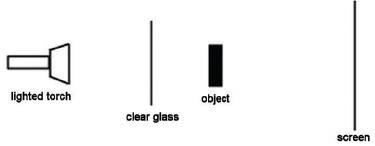A shadow was formed on the screen.

Q. How could the shadow on the screen be enlarged?

Solution:
QUESTION: 22

Which of the following parts of the bulb are made of conductors of electricity?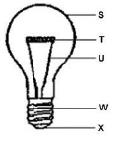Solution:
QUESTION: 23

Study the circuit below.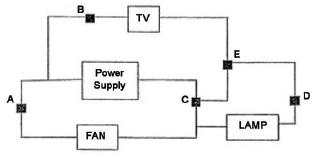The switches at points A, B, C, D and E were closed and all 3 electrical appliances were working.
Q. Opening the switch at point  would allow both the TV and the lamp to work.

Solution:
QUESTION: 24

What is function of the components shown below?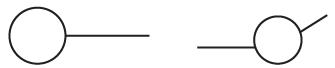Solution:
QUESTION: 25

On which property of magnet does the design of the mariner’s compass is based?

Solution:
QUESTION: 26

The diagram below shows a compass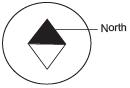Q. Two compasses are placed near a bar magnet. Which one of the following diagrams shows the correct positions of the needles?

Solution:

A freely suspended bar magnet always points in northsouth direction. The end that points towards north direction is the north pole of the magnet while the end that points towards south direction is the south pole of the magnet.

QUESTION: 27

This set of elements produce strong magnets?

Solution:
QUESTION: 28

Select the option the letters of which, on reshuffling, will give the term given to describe water seepage into the ground.

Solution:

Infiltration is the process by which water on the ground surface enters the soil. It is commonly used in both hydrology and soil sciences. The infiltration capacity is defined as the maximum rate of infiltration.

QUESTION: 29

Which of the following actions will help in reducing pollution?
1. Restricting smoking in public areas
2. Treatment of sewage to remove human waste
3. Using unleaded petrol in cars
4. Spraying pesticides on paints to kill pests

Solution:
QUESTION: 30

Which of the following is not a form of precipitation?

Solution: